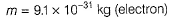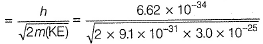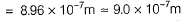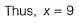Courses

# Dual Nature Of Matter & Heisenberg Uncertainty Principle

## 16 Questions MCQ Test Chemistry for JEE | Dual Nature Of Matter & Heisenberg Uncertainty Principle

Description
This mock test of Dual Nature Of Matter & Heisenberg Uncertainty Principle for JEE helps you for every JEE entrance exam. This contains 16 Multiple Choice Questions for JEE Dual Nature Of Matter & Heisenberg Uncertainty Principle (mcq) to study with solutions a complete question bank. The solved questions answers in this Dual Nature Of Matter & Heisenberg Uncertainty Principle quiz give you a good mix of easy questions and tough questions. JEE students definitely take this Dual Nature Of Matter & Heisenberg Uncertainty Principle exercise for a better result in the exam. You can find other Dual Nature Of Matter & Heisenberg Uncertainty Principle extra questions, long questions & short questions for JEE on EduRev as well by searching above.
QUESTION: 1

### Direction (Q. Nos. 1-10) This section contains 10 multiple choice questions. Each question has four choices (a), (b), (c) and (d), out of which ONLY ONE option is correct. Which of the following properties of atom could be explained correctly by Thomson Model of atom?

Solution:

According to Thomson model of atom, the mass of the atom is assumed to be uniformly distributed over the atom. This model was able to explain the overall neutrality of the atom.

QUESTION: 2

### The threshold frequency v0 for a metal is 7.0 x 1014 s-1. Radiation of frequency v = 1.0 x 1015 s-1 hits the metal. Kinetic energy of the emitted electron is

Solution:

By photoelectric effect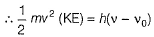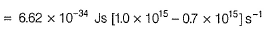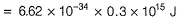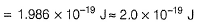QUESTION: 3

### Which of the following rules/principles is responsible to rule out the existence of definite paths or trajectories of electrons?

Solution:

By Heisenberg’s uncertainty principle, it is impossible to determine position and momentum simultaneously with same accuracy. Thus, existence of definite path is ruled out.

QUESTION: 4

In an atom, an electron is moving with a speed of 600 ms-1 with an accuracy of 0.005%. Certainty with which the position of the electron can be located is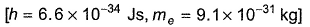[AIEEE 2009]

Solution:

By Heisenberg’s uncertainty principle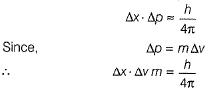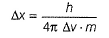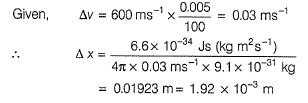QUESTION: 5

The wavelength (in nanometer) associated with a proton (mass = 1.67 x 10-27 kg atom-1) at the velocity of 1.0 x 10-3 ms-1 is

Solution:

By de-Broglie’s equation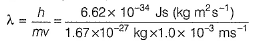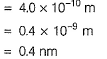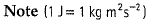QUESTION: 6

Statement I : It is impossible to determine position and momentum of the moving electron simultaneously with same accuracy.
Statement II : The path of an electron in an atom is clearly defined.

Solution:

Statement 1 is uncertainty principle, according to which we can't locate a moving electron accurately at any instant, which implies there must me certain uncertainty in its path, as path basically gives us the position of electron at various instances.

QUESTION: 7

If the photon of the wavelength 150 pm strikes an atom and one of its inner bound electrons is ejected out with a velocity of 1.5 x 107 ms-1, then binding energy by which electron is bound to nucleus is

Solution:

Binding energy is the minimum energy to which electron is bound to nucleus or which is required to eject the electron.
Thus, binding energy = - threshold energy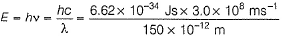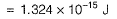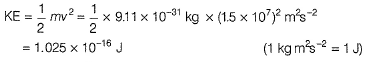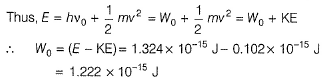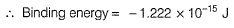QUESTION: 8

The position of both, an electron and a helium atom is known within 1.0 mm. Further more the momentum of the electron is known within 5.0 x 10-26 kg ms-1. The minimum uncertainty in the measurement of the momentum of the helium atom is

Solution: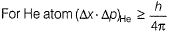and for electron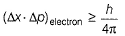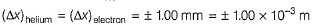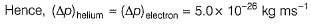QUESTION: 9

The wavelength of a neutron with a translatory kinetic energy equal to kT at 300 K is

Solution:

Kinetic energy = kT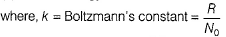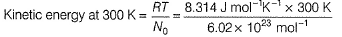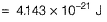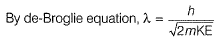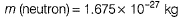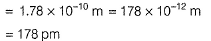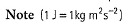QUESTION: 10

Frequency of a matter wave is equal to

Solution:

By de-Broglie equation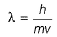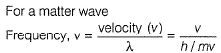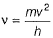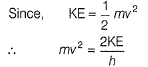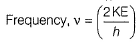QUESTION: 11

Direction (Q. Nos. 11 and 12) This section contains a paragraph, wach describing  theory, experiments, data etc. three Questions related to paragraph have been  given.Each question have only one correct answer among the four given  ptions  (a),(b),(c),(d)

Photoelectric effect can be expressed in terms of the following graph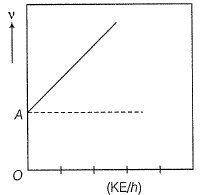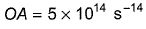Q. What is work function for this photoelectric emission of electrons?

Solution:

Photoelectric effect is represented by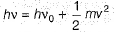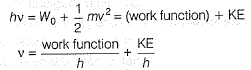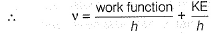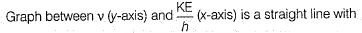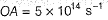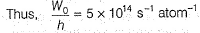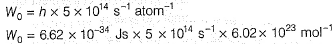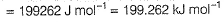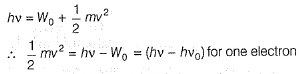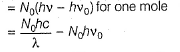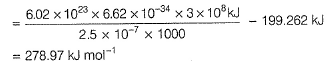QUESTION: 12

Photoelectric effect can be expressed in terms of the following graphQ. Kinetic energy imparted to the moving electron at a wavelength of 2.5 x 10-7 m is

Solution:

Photoelectric effect is represented byQUESTION: 13

Direction (Q. Nos. 13) Choice the correct combination of elements and column I and coloumn II are given as option (a), (b), (c) and (d), out of which ONE option is correct.

Q.

Neon gas is generally used in sign boards. It emits radiations at wavelength 616 nm.
Match the values derived given in Column II with the corresponding parameter given in Column I.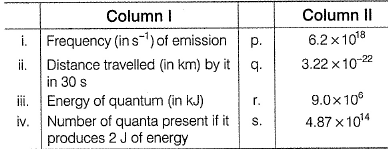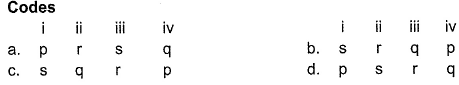Solution: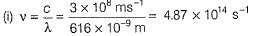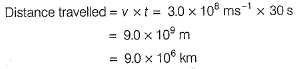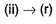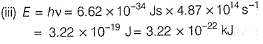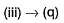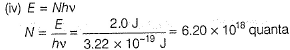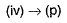*Answer can only contain numeric values
QUESTION: 14

Direction (Q. Nos. 14 - 16) This section contains 2 questions. when worked out will result in an integer from 0 to 9 (both inclusive)

Q.

The work function (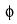) of some metals is listed below. The number of metals which will show photoelectric effect when light of 300 nm wavelength falls on the metal i s ........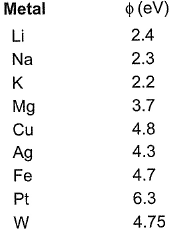Solution: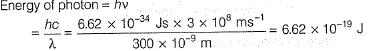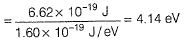Thus, any metal with energy less than 4.14 eV will emit photons thus, showing photoelectric effect. (Li, Na, K, Mg)

*Answer can only contain numeric values
QUESTION: 15

If the uncertainties in the measurements of position and momentum are equal, calculate the uncertainty in the measurement of velocity (in ms-1) of particle of mass 1.21 x 10-18 kg

Solution:

By Heisenberg’s uncertainty principle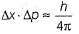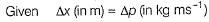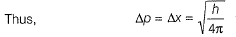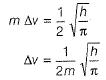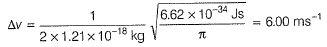*Answer can only contain numeric values
QUESTION: 16

Wavelength associated with an electron having KE 3.0 x 10-25 J is x *10-7 m.

Q. What is the value of x?

Solution:

By de-Broglie equation